# Concept 4 – Proportional Relationships

Week 8 - Proportionality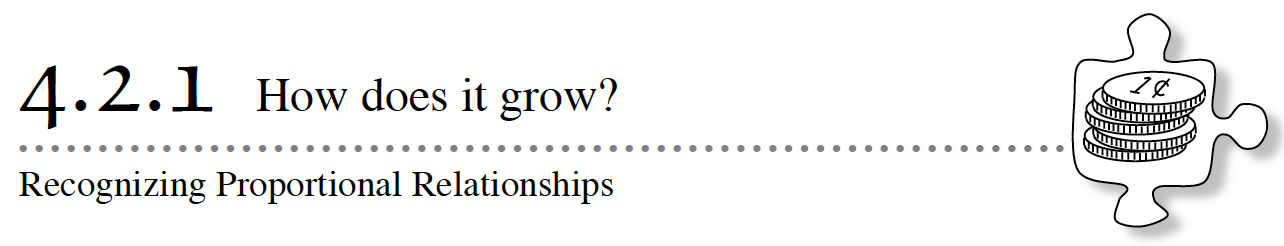Grocery stores often advertise special prices for fruits and vegetables that are in season.  You might see a sign that says, “Special Today!  Buy 2 pounds of apples for \$1.29!”  How would you use that information to predict how much you need to pay if you want to buy six pounds of apples?  Or just 1 pound of apples?  The way that the cost of apples grows or shrinks allows you to use a variety of different strategies to predict and estimate prices for different amounts of apples.  In this section, you will explore different kinds of growth patterns.  You will use those patterns to develop strategies for making predictions and deciding if answers are reasonable.

How are the entries in the table related?
Can I double the values?
What patterns can I see in a graph?

4-21. COLLEGE FUND

Five years ago, Gustavo’s grandmother put some money in a college savings account for him on his birthday.  The account pays simple interest, and now, after five years, the account is worth \$500.  Gustavo predicts that if he does not deposit or withdraw any money, then the account balance will be \$1000 five years from now.

1. How do you think Gustavo made his prediction
2. Do you agree with Gustavo’s reasoning?  Explain why or why not.

4-22. Last week, Gustavo got his bank statement in the mail.  He was surprised to see a graph that showed that, although his balance was growing at a steady rate, the bank predicted that in five years his account balance would be only \$600.  “What is going on?” he wondered.  “Why isn’t my money growing the way I thought it would?”

With your team, discuss how much Gustavo’s account appears to be growing every year.  Why might his account be growing in a different way than he expected?  Be ready to share your ideas.

4-23. Gustavo decided to look more carefully at his balances for the last few years to see if the bank’s prediction might be a mistake.  He put together the table below.

 Time since Original Deposit (in yrs) 2 3 4 5 Bank Balance (in dollars) 440 460 480 500
1. How has Gustavo’s bank balance been growing?
2. Does Gustavo’s money seem to be doubling as the number of years doubles?  Explain your reasoning.

4-24. Once Gustavo saw the balances written in a table, he decided to take a closer look at the graph from the bank to see if he could figure out where he made the mistake in his prediction.  Find the graph below on the Lesson 4.2.1 Resource Page or use the 4-24 Student eTool (Desmos).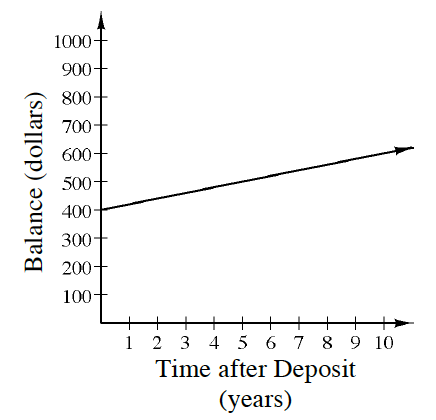• There is additional information about Gustavo’s account that you can tell from the graph.  For example, what was his starting balance?  How much does it grow in 5 years?
• Gustavo had assumed his money would double after 10 years.  What would the graph look like if that were true?  Using a different color, add a line to the graph that represents what Gustavo was thinking.
• Is it possible that Gustavo’s account could have had \$0 in it in Year 0?  Why or why not?

4-25. FOR THE BIRDS

When filling her bird feeder, Sonja noticed that she paid \$27 for four pounds of bulk birdseed.  “Next time, I’m going to buy 8 pounds instead so I can make it through the spring.  That should cost \$54.”

1. Does Sonja’s assumption that doubling the amount of birdseed would double the price make sense?  Why or why not?  How much would you predict that 2 pounds of birdseed would cost?
2. To check her assumption, she found a receipt for 1 pound of birdseed.  She decided to make a table, which is started below.  Copy and complete her table or use the 4-25 Student eTool (Desmos).
 Pounds 0 1 2 3 4 5 6 8 Cost \$6.75 \$27
3. How do the amounts in the table grow?
4. Does the table confirm Sonja’s doubling relationship?  Give two examples from the table that show how doubling the pounds will double the cost.

4-26. What makes Sonja’s birdseed situation (problem 4-25) different from Gustavo’s college fund situation (problem 4-21)?  Why does doubling work for one situation but not in the other?  Consider this as you examine the graphs below.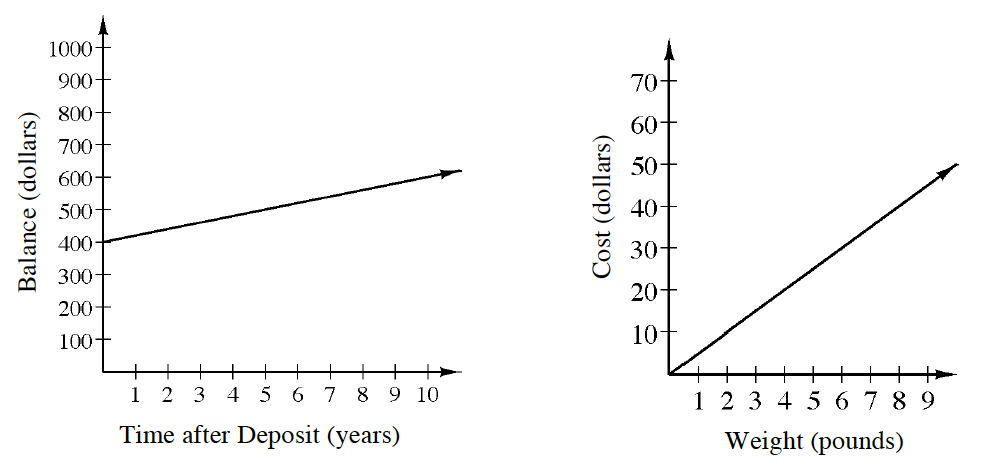a. Describe how each graph is the same.

b. Describe what makes each graph different.

c. How do the differences explain why doubling works in one situation and not in the other?  Generalize why doubling works in one situation and not in another.

d. The pattern of growth in Sonja’s example of buying birdseed is an example of a proportional relationship.  In a proportional relationship, if one quantity is multiplied by a scale factor, the other is scaled by the same amount.  Gustavo’s bank account is not proportional, because it grows differently; when the number of years doubled, his balance did not. Work with your team to list other characteristics of proportional relationships, based on Sonja’s and Gustavo’s examples.  Be as specific as possible.

4-27. IS IT PROPORTIONAL?

When you are making a prediction, it is important to be able to recognize whether a relationship is proportional or not.

Your Task: Work with your team to read each new situation below.  Decide whether you think the relationship described is proportional or non-proportional and justify your reasoning.  Be prepared to share your decisions and justifications with the class.

1. Carlos wants to buy some new video games.  Each game he buys costs him \$36.  Is the relationship between the number of games Carlos buys and the total price proportional?
2. A single ticket to a concert costs \$56, while buying five tickets costs \$250.  Is the relationship between the number of tickets bought and the total price proportional?
3. Vu is four years older than his sister.  Is the relationship between Vu and his sister’s age proportional?
4. Janna runs at a steady pace of 7 minutes per mile.  Is the relationship between the number of miles she ran and the distance she covered proportional?
5. Carl just bought a music player and plans to load 50 songs each week.  Is the relationship between the number of weeks after Carl bought the music player and the number of songs on his player proportional?
6. Anna has a new video game.  It takes her five hours of playing the game to master level one.  After so much time, Anna understands the game better and it only takes her three hours of playing the game to master level two.  Is the number of hours played and the game level proportional?

Assignment: 4.2.1 Homework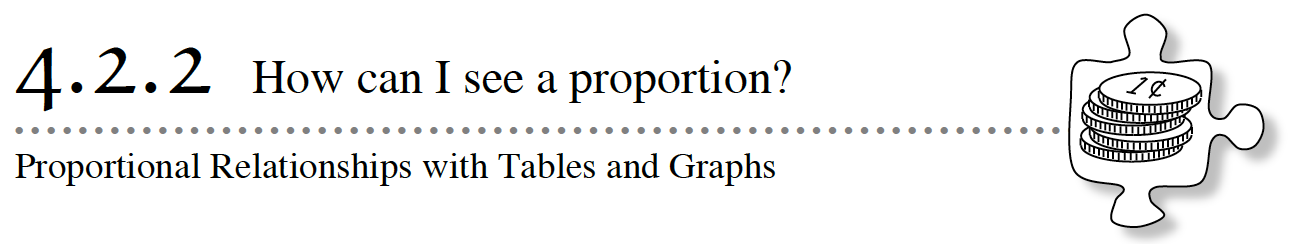In Lesson 4.2.1, you learned that you could identify proportional relationships by looking for a constant multiplier.  In fact, you have already seen a relationship with a constant multiplier in this course.  Today you will revisit the earlier situation that contains a proportional relationship.

Assignment: 4.2.2 Homework

Week 9 - Unit Rate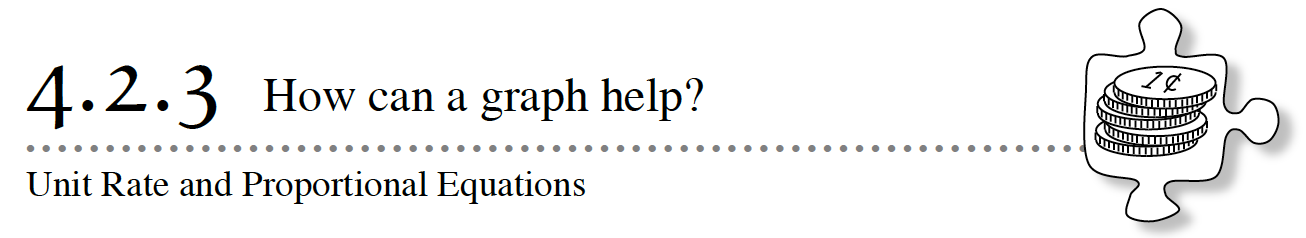Proportional relationships can be identified in both tables and graphs.  Today you will have an opportunity to take a closer look at how graphs and tables for proportional relationships can help you organize your work to find any missing value quickly and easily.

4-46. Robert’s new hybrid car has a gas tank that holds 12 gallons of gas.  When the tank is full, he can drive 420 miles.  Assume that his car uses gas at a steady rate.

1. Is the relationship between the number of gallons of gas used and the number of miles that can be driven proportional?  For example, does it change like Sonja’s birdseed prediction, or is it more like Gustavo’s college savings?  Explain how you know.
2. Show how much gas Robert’s car will use at various distances by copying and completing the table below.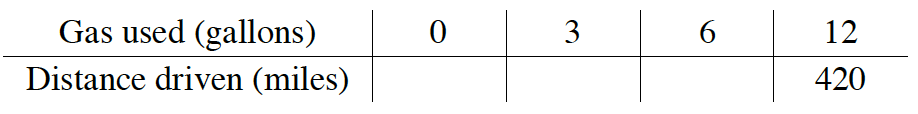3.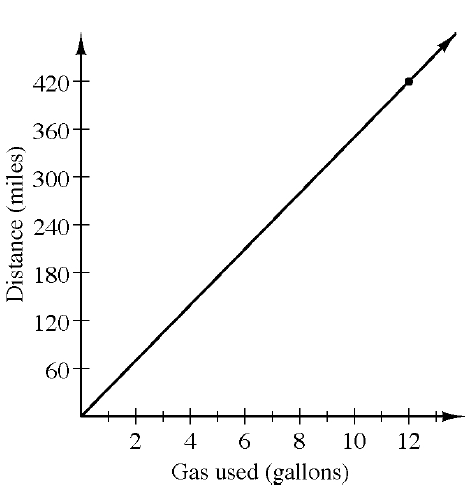Robert decided to graph the situation, as shown below.  The distance Robert can travel using one gallon of gas is called the unit rate.  Use Robert’s graph to predict how far he can drive using one gallon of gas.  That is, find his unit rate.
4. While a graph is a useful tool for estimating, it is often difficult to find an exact answer on a graph.  What is significant about the point labeled (1, y)?  How can you calculate y?
5. Use the table in part (b) and your result in part (d) to find Robert’s unit rate.
6. Work with your team to write the equation to find the exact number of miles Robert can drive with any number of gallons of gas.  Be prepared to share your strategy.
7. Use your equation to find out how many gallons of gas Robert will need to drive 287 miles.  .

4-47. THE YOGURT SHOP

Jell E. Bean owns the local frozen yogurt shop.  At her store, customers serve themselves a bowl of frozen yogurt and top it with chocolate chips, frozen raspberries, and any of the different treats available.  Customers must then weigh their creations and are charged by the weight of their bowls.

Jell E. Bean charges \$32 for five pounds of dessert, but not many people buy that much frozen yogurt.  She needs you to help her figure out how much to charge her customers.  She has customers that are young children who buy only a small amount of yogurt as well as large groups that come in and pay for everyone’s yogurt together.

1. Is it reasonable to assume that the weight of the yogurt is proportional to its cost?  How can you tell?
2. Assuming it is proportional, make a table that lists the price for at least ten different weights of yogurt.  Be sure to include at least three weights that are not whole numbers.
3. What is the unit rate of the yogurt?  (Stores often call this the unit price.)  Use the unit rate to write an equation that Jell E. Bean can use to calculate the amount any customer will pay.
4. If Jell E. Bean decided to start charging \$0.50 for each cup before her customers started filling it with yogurt and toppings, could you use the same equation to find the new prices?  Why or why not?

4-48. Lexie claims that she can send 14 text messages in 22 minutes.  Her teammates Kenny and Esther are trying to predict how many text messages Lexie can send in a 55‑minute lunch period if she keeps going at the same rate.

1. Is the relationship between the number of text messages and time in minutes proportional?  Why or why not?
2. Kenny represented the situation using the table shown below.  Explain Kenny’s strategy for using the table.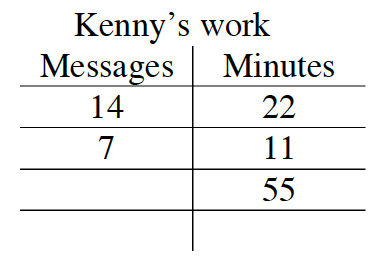3. Esther wants to solve the problem using an equation.  Help her write an equation to determine how many text messages Lexie could send in any number of minutes.
4. Find the missing value in Kenny’s table.
5. Solve Esther’s equation.  Will she get the same answer as Kenny?
6. What is Lexie’s unit rate?  That is, how many text messages can she send in 1 minute?

4-49. Additional Challenge: Use your reasoning skills to compute each unit rate (the price per pound).

1. \$4.20 for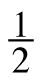pound of cheese
2. \$1.50 for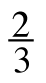pound of bananas
3. \$6.00 for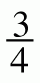pound of deli roast beef
4. \$7.50 for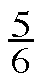pound of sliced turkey

Assignment: 4.2.3 Homework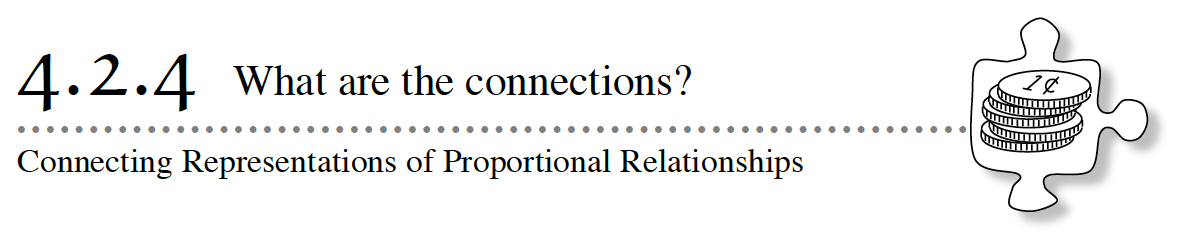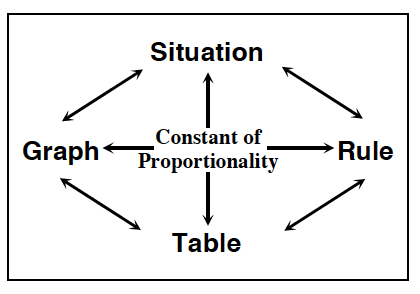In the previous lessons, you studied different ways to represent proportional relationships.  You organized information into tables and graphs.  You also wrote equations modeling the proportional relationships.  Proportional relationship equations are of the form  y = kx, where  k  is the constant of proportionality.  Today you will find connections between different representations of the same proportional relationship, explore each representation more deeply, and learn shorter ways to go from one representation to another.  As you work today, keep these questions in mind:

How can you see growth in the rule?

How do you know your rule is correct?

What does the representation tell you?

What are the connections between the representations?

4-55. Graeme earns \$4.23 for each half hour that he works.  How much money does he earn during a given amount of time?

1. Represent this situation using a table
2. What is the constant of proportionality (or the unit rate)?  How can you find it from a table?
3. How can you use a table to determine if a relationship is proportional?

4-56. Jamie ran 9.3 miles in 1.5 hours.  How far can she run in a given amount of time, if she runs at a constant rate?

1. Represent this situation with a graph.
2. What is the constant of proportionality?  How can you find it on a graph?
3. How can you use a graph to determine if a relationship is proportional?

4-57. A recipe calls for 2cups of of flour to make two regular batches of cookies.  Shiloh needs to make multiple batches of cookies.

1. Represent this situation with an equation.
2. What is the constant of proportionality?  How can you identify it in an equation?
3. How can you use an equation to determine if a relationship is proportional?

Assignment: 4.2.4 Homework

Week 10 - Proportional Relationships1. Work with a partner to answer Alex’s question.

1. Are Alex’s total earnings proportional to the number of weeks he worked?  How do you know?• Why is it important for you to know that miles are proportional to the gallons used?

• Describe the approach you used to complete the table.

• What is the value of the constant? Explain how the constant was determined.1. For which team is distance proportional to time? Explain your reasoning.

1. Explain how you know the distance for the other team is not proportional to time.

1. If the race were 2.5 miles long, which team would win? Explain.

1. If the race were 3.5 miles long, which team would win? Explain.

1. If the race were 4.5 miles long, which team would win? Explain.

1. For what length of race would it be better to be on Team B than Team A? Explain.

1. Using this relationship, if the members on the team ran for 10 hours, how far would each member run on each team?

1. Will there always be a winning team, no matter what the length of the course? Why or Why not?

1. If the race were 12 miles long, which team should you choose to be on if you wish to win? Why would you choose this team?

1. How much sooner would you finish on that team compared to the other team?Portion Web Practice